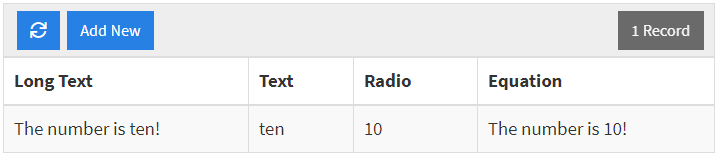This article provides details on our Text Functions that are available for use with the Equation Field.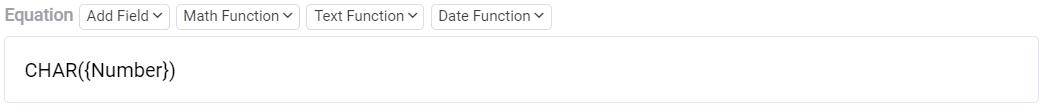The CHAR function generates a character from a numeric value. The result is going to be based on the corresponding character of the numeric input read as an int ASCII code. The input should be a value from 0-255. For a character guide, please click here.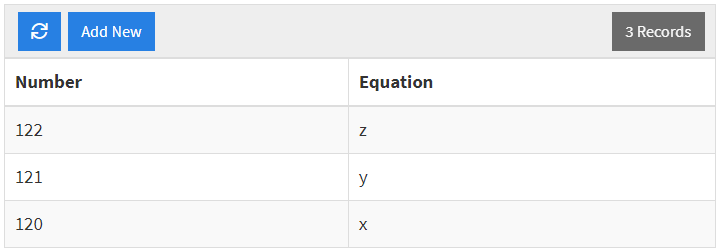CONCAT: Use Case 1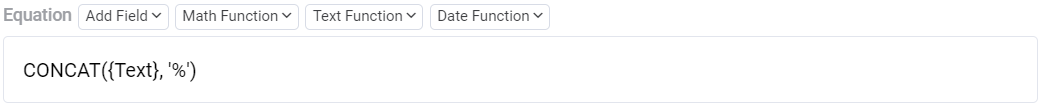CONCAT: Use Case 2The CONCAT function concatenates the given comma-separated inputs. This function requires at least one value, however, two or more should be present for there to a difference between the input and the produced value. There are two main types of use cases for this function. The first is a single field combined with static text. The second is multiple fields combined with static text.

Taking a closer look at Use Case 1, we can see that (a) is the field and (b) is the static text. When adding text into your function, ensure that it is within single quotes.Now, looking at Use Case 2, we can see that (a) and (c) are the fields and (b) is the static text. This example shows the use of CHAR to place a space between the two fields. No more than 254 total fields and static text instances can be used within the CONCAT function.Here are some examples of outputs for the two use cases we have overviewed.

CONCAT: Use Case 1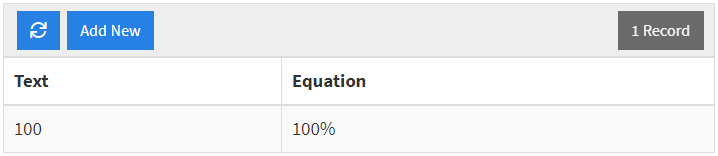CONCAT: Use Case 2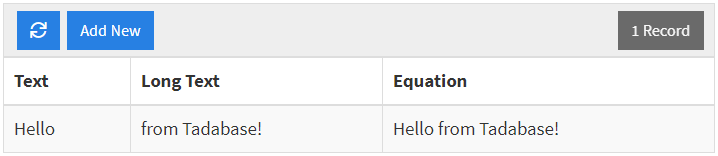The LOWER function generates the lowercase representation of your given values. The expected input for this function is a single field.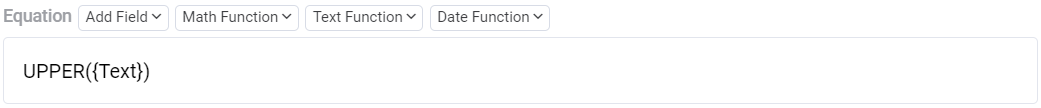The UPPER function generates the uppercase representation of your given values. The expected input for this function is a single field.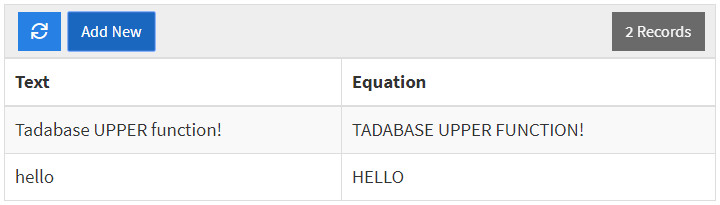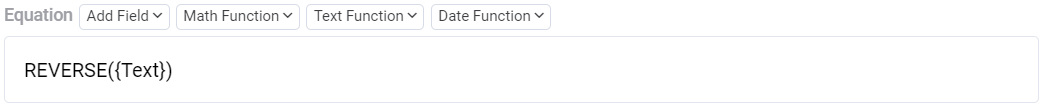The REVERSE function generates the reverse representation of your given values. The expected input for this function is a single field.The LENGTH function generates a numeric representation of the number of characters in your given values. The expected input for this function is a single field.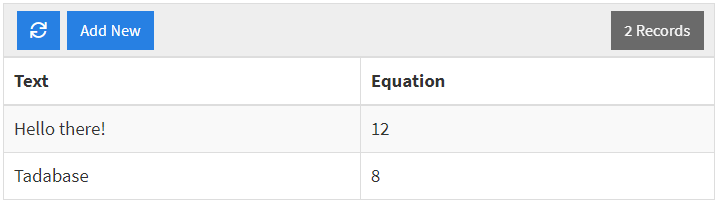The REPLACE function replaces a defined set of characters with another defined set of characters within your given values. There are three expected inputs for this type of field. Referencing the image below, (a) is the value where the place will be performed within, (b) is the text to replace, and (c) is the text that will replace the prior value.Below are some results corresponding to the configurations defined above.